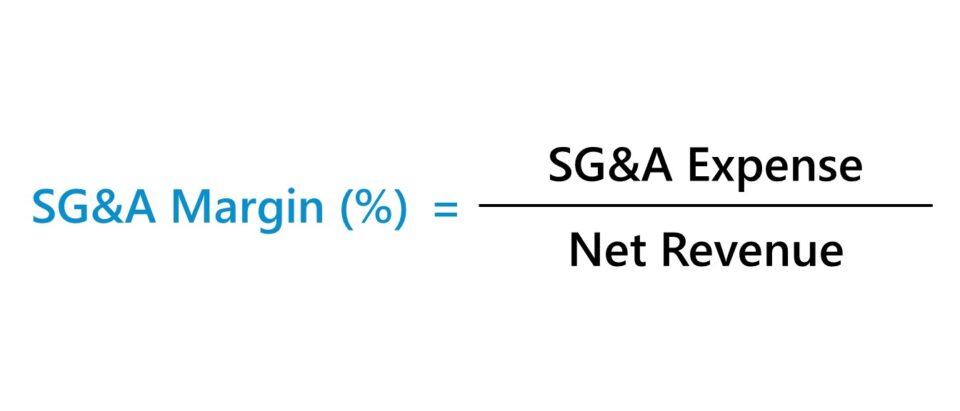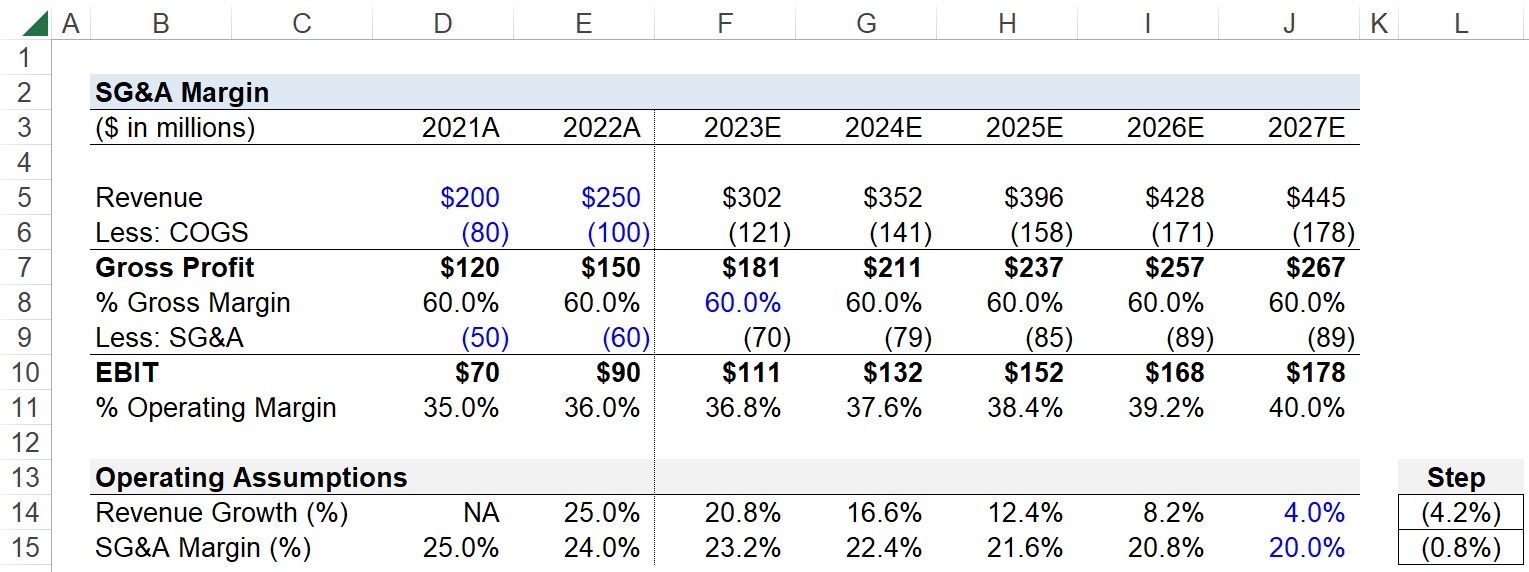Welcome to Wall Street Prep! Use code at checkout for 15% off.# SG&A Margin

Guide to Understanding the SG&A Margin## How to Calculate SG&A Margin?

The SG&A margin describes the relationship between a company’s selling, general and administrative costs and the amount of revenue generated in the corresponding period.

• SG&A Expense: SG&A stands for “selling, general & administrative” and is a catch-all categorization capturing indirect costs that do not meet the criteria to be recognized as cost of goods sold (COGS); i.e. SG&A consists of operating costs not directly tied to revenue generation.
• Net Revenue: Net revenue refers to the monetary value obtained by a company from the sale of its products or services to customers over a specified period. The net revenue metric is calculated by taking a company’s gross revenue and adjusting for discounts, returns, and sales allowances.

On the income statement, the first adjustment to net revenue – i.e. the “top line” – is the cost of goods sold (COGS) line item, which captures the operating costs incurred that are directly related to a company’s efforts to generate revenue.

From the gross profit line item, which is equal to revenue minus COGS, the next adjustment is for indirect operating costs, or SG&A.

Upon deducting a company’s SG&A from gross profit – assuming there are no other operating expenses – the resulting profit metric is operating income (EBIT).

Unlike a company’s COGS, the incurred SG&A expense is not directly tied to its revenue generation. Instead, SG&A represents the indirect costs that stem from day-to-day operations, such as purchasing office supplies, overhead costs, and rent.

The process of calculating a company’s SG&A margin can be broken up into three steps:

• Step 1. Obtain the Net Revenue and SG&A Figures from the Income Statement
• Step 2. Divide SG&A by Net Revenue
• Step 3. Convert into a Percentage by Multiplying the Resulting Figure in Decimal Form by 100

## SG&A Margin Formula

The formula to calculate the SG&A margin is as follows.

SG&A Margin (%) = SG&A Expense ÷ Net Revenue

In order for the SG&A margin to be meaningful, the company’s operating income (EBIT) must be positive, i.e. there are remaining profits after deducting COGS and SG&A from revenue.

In addition, a negative sign must be placed in front of the formula if SG&A was entered as a negative integer as part of the sign convention used in the financial model; otherwise, the returned margin will be a negative percentage.

## What is a Good SG&A Ratio?

Conceptually, the SG&A ratio measures the percentage of each dollar of revenue earned by a company allocated to SG&A.

For instance, a 25% SG&A ratio implies that for each dollar of revenue brought in, a quarter of it is spent on SG&A expenses.

As a general rule, the lower the SG&A margin, the better. However, comparisons must be made relative to the industry within which the company operates, as the average benchmark varies significantly by industry.

The SG&A margin ratio can be informative in terms of understanding a company’s cost structure.

• Low SG&A Ratio: Lower Percentage of Indirect Costs in Cost Structure
• High SG&A Ratio: Higher Percentage of Indirect Costs in Cost Structure

That said, the SG&A margin ratio can be a useful tool for understanding where a company’s revenue is spent, i.e. the concentration of costs in the overall business model, which ultimately determines a company’s profitability.

## SG&A Margin and Operating Profit Margin

The SG&A margin is calculated by dividing a company’s SG&A by its revenue. In contrast, the operating profit margin (or “EBIT margin”) is calculated by dividing a company’s operating income by revenue.

Operating Profit Margin (%) = EBIT ÷ Net Revenue

Where:

• EBIT = Gross Profit – SG&A

Therefore, the SG&A margin and operating profit margin are inversely related.

Operating Profit Margin (%) = 1  SG&A Margin (%)
SG&A Margin (%) = 1  Operating Profit Margin (%)

## How to Forecast SG&A Expense?

For forecasting, the most common method is to project SG&A expense as a percentage of revenue.

Forecasted SG&A Expense = SG&A % Revenue Assumption × Revenue

The historical SG&A margin is first calculated before assessing the trend of the ratio.

• Historical Average: If the SG&A ratio remains relatively stable – which tends to be the case for mature companies, since SG&A comprises fixed costs – the historical average can be referenced and followed for future periods.
• Trend Analysis: But if the SG&A ratio has moved either upward or downward, the trend can be followed until a normalized margin is reached, i.e. to a sustainable percentage aligned with the industry average.

If SG&A is the only operating expense, the operating profit margin could technically be used as the driver of the projection. However, directly projecting EBIT is seldom done in practice and is generally not recommended, especially for more complex models.

## SG&A Margin Calculator

We’ll now move on to a modeling exercise, which you can access by filling out the form below.Submitting...

## 1. Historical SG&A Margin Calculation Example

Suppose you’re tasked with building a five-year forecast of a company’s SG&A and operating profit (EBIT) using the following historical income statement data.

Historical Data 2021A 2022A
Revenue \$200 million \$250 million
Less: COGS (80 million) (100 million)
Gross Profit \$120 million \$150 million
Less: SG&A (\$50 million) (\$60 million)
EBIT \$70 million \$90 million

Historically, the company’s gross margin was 60% in both periods, while its SG&A margin was 25% and 24% in 2021 and 2022, respectively.

For our SG&A margin assumption, we’ll assume that given the recent decline in the ratio (and references to comparable mature companies), the SG&A margin in 2027 will be 20%.

## 2. SG&A Expense Projection

In the next section, we’ll project our company’s SG&A expense (and operating margin) over the five-year forecast period.

For our revenue assumptions, we’ll assume the growth rate will decline to 4.0% by the end of 2027, while the gross margin remains fixed at 60% throughout the forecast.

Using a step function, we’ll enter our final year SG&A margin in our operating assumptions section, so that the percentage declines in equal increments starting from the end of 2022.

Since the SG&A margin is declining, the impact on EBIT (and thus the company’s operating profit margin) is positive.

For each forecast period, we’ll multiply our SG&A margin assumption by the projected revenue in the same period, which results in our projected SG&A expense amounts.

The EBIT line item is equal to the gross profit subtracted by SG&A, so we’ve indirectly forecasted our company’s EBIT, which can be divided by revenue, to arrive at the operating profit margin for each period.

In conclusion, we can see our company’s SG&A margin declined from 24% initially to 20% by the end of the forecast, whereas the operating profit margin increased from 36% to 40% across the same time horizon.Step-by-Step Online Course

### Everything You Need To Master Financial Modeling

Enroll in The Premium Package: Learn Financial Statement Modeling, DCF, M&A, LBO and Comps. The same training program used at top investment banks.

Inline Feedbacks